# Linear motion

### Guest Account

Attempt Form Three Physics Questions
Guest Account

# Linear motion

Introduction
- It is the study of motion. It is divided into two.
• Kinematics
• Dynamics
- In kinematics, forces causing motion are disregarded while dynamics deals with motion of objects and the forces causing them.

### I. Displacement

- Distance moved by a body in a specified direction is called displacement. It is denoted by letter‘s’ and has both magnitude and direction. Distance is the movement from one point to another. The Si unit for displacement is the metre (m).

### II. Speed

- This is the distance covered per unit time. Speed = distance covered / time taken. Distance is a scalar quantity since it has magnitude only. The SI unit for speed is metres per second (m/s or ms-1)
Average speed = total distance covered / total time taken.
- Another unit for speed used is Km/h.

Example 1
A body covers a distance of 10m in 4 seconds. It rests for 10 seconds and finally covers a distance of 90m in 60 seconds. Calculate the average speed.
Solution

Total distance covered = 10 + 90 = 100m
Total time taken = 4 + 10 + 6 = 20 seconds
Therefore average speed = 100/20 = 5m/s

Example 2
Calculate the distance in metres covered by a body moving with a uniform speed of 180 km/h in 30 seconds.
Solution

Distance covered = speed × time
= 180 × 1000/60 × 60 = 50m/s
= 50 × 30
= 1,500m

Example 3
Calculate the time in seconds taken a by body moving with a uniform speed of 360km/h to cover a distance of 3,000 km?
Solution

Speed: 360 km/h = 360 × 1000/60 × 60=100m/s
Time = distance/speed
3000 × 1000/100
= 30,000 seconds.

### III. Velocity

- This is the change of displacement per unit time. It is a vector quantity.
Velocity=change in displacement/total time taken.
The SI units for velocity are m/s.
Example 4
A man runs 800m due North in 100 seconds, followed by 400m due South in 80 seconds. Calculate,
a. His average speed.
b. His average velocity.
c. His change in velocity for the whole journey

Solution

1. Average speed: total distance travelled/total time taken.
= 800 + 400/100 + 80
= 1200/180
= 6.67m/s
2. Average velocity: total displacement/total time.
= 800 - 400/180
= 400/180
= 2.22 m/s due North
3. Change in velocity=final-initial velocity
= (800/100) - (400-80)
= 8 - 5
= 3m/s due North

Example 5
A tennis ball hits a vertical wall at a velocity of 10m/s and bounces off at the same velocity. Determine the change in velocity.
Solution

Initial velocity (u) = -10m/s
Final velocity (v) = 10m/s
Therefore change in velocity = v-u
= 10- (-10)
= 20m/s

### IV. Acceleration

- This is the change of velocity per unit time. It is a vector quantity symbolized by ‘a’.
Acceleration 'a' = change in velocity/time taken= v - u/t.
- The SI units for acceleration are m/s2.

Example 6
The velocity of a body increases from 72 km/h to 144 km/h in 10 seconds. Calculate its acceleration.
Solution

Initial velocity= 72 km/h = 20m/s
Final velocity= 144 km/h = 40m/s
Therefore 'a' = v - u/t
= 40 - 20/10
= 2m/s2

Example 7
A car is brought to rest from 180km/h in 20 seconds. What is its retardation?
Solution

Initial velocity = 180km/h = 50m/s
Final velocity= 0 m/s
A = v - u/t = 0 - 50/20
= -2.5 m/s2
Hence retardation is 2.5 m/s2

## Motion Graphs

### Distance-time Graphs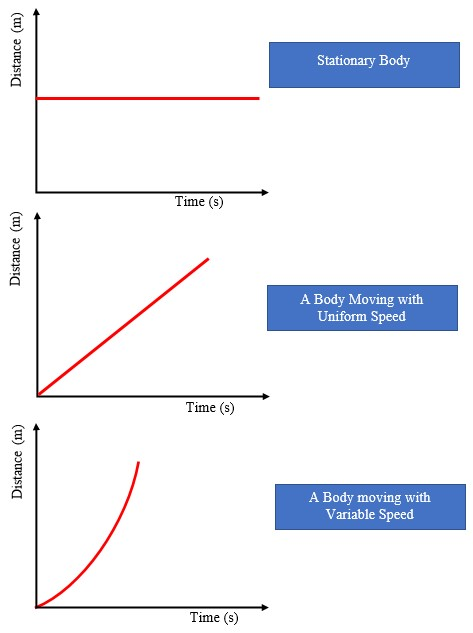Area under velocity-time graph
Consider a body with uniform or constant acceleration for time ‘t’ seconds;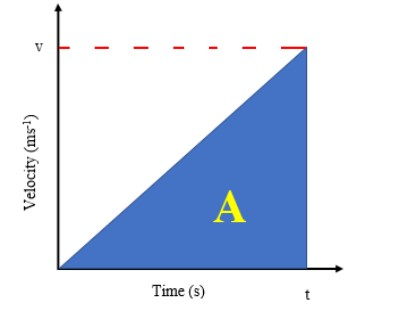Distance travelled= average velocity × t
= (0 + v/2) × t
= ½vt
This is equivalent to the area under the graph. The area under velocity-time graph gives the distance covered by the body under 't' seconds.
Example 8
A car starts from rest and attains a velocity of 72km/h in 10 seconds. It travels at this velocity for 5 seconds and then decelerates to stop after another 6 seconds. Draw a velocity-time graph for this motion. From the graph;
i. Calculate the total distance moved by the car.
ii. Find the acceleration of the car at each stage.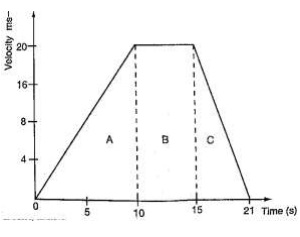Solution

1. a. From the graph, total distance covered= area of (A + B + C)
= (½ × 10 × 20) + (½ × 6 × 20) + (5 × 20)
= 100 + 60 + 100
= 260m
Also the area of the trapezium gives the same result.

2. Acceleration= gradient of the graph
Stage A gradient= (20-0)/(10-0) = 2 m/s2
Stage b gradient= (20-20)/(15-10) = 0 m/s2
Stage c gradient= (0-20)/(21-15) = -3.33 m/s2

#### Using a ticker-timer to measure speed, velocity and acceleration.

It will be noted that the dots pulled at different velocities will be as follows: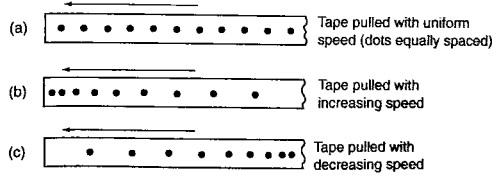- Most ticker-timers operate at a frequency of 50Hz i.e. 50 cycles per second hence they make 50 dots per second. Time interval between two consecutive dots is given as,
1/50 seconds= 0.02 seconds. This time is called a tick.
The distance is measured in ten-tick intervals hence time becomes 10×0.02= 0.2 seconds.

Example 9
a. A tape is pulled steadily through a ticker-timer of frequency 50 Hz. Given the outcome below, calculate the velocity with which the tape is pulled.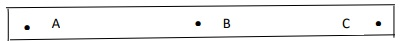Solution

Distance between two consecutive dots= 5cm
Frequency of the ticker-timer=50Hz
Time taken between two consecutive dots=1/50=0.02 seconds
Therefore, velocity of tape=5/0.02= 250 cm/s

Example 10
The tape below was produced by a ticker-timer with a frequency of 100Hz. Find the acceleration of the object which was pulling the tape.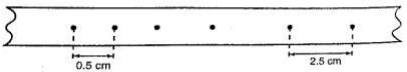Solution

Time between successive dots = 1/100 = 0.01 seconds
Initial velocity (u) 0.5/0.01 = 50 cm/s
Final velocity (v) 2.5/0.01 = 250 cm/s
Time taken= 4 × 0.01 = 0.04 seconds
Therefore, acceleration= v - u/t = 250 - 50/0.04 = 5,000 cm/s2

### Equations of linear motion

The following equations are applied for uniformly accelerated motion;
1. v = u + at
2. s = ut + ½ at2
3. v2= u2 + 2as
Example 11
A body moving with uniform acceleration of 10 m/s2 covers a distance of 320 m.
If its initial velocity was 60 m/s. Calculate its final velocity.
Solution

V2 = u2 +2as
= (60) + 2 × 10 × 320
= 3600 + 6400
= 10,000
Therefore v = (10,000)1/2
v= 100m/s

Example 12
A body whose initial velocity is 30 m/s moves with a constant retardation of 3m/s. Calculate the time taken for the body to come to rest.
Solution

v = u + at
0 = 30-3t
30 = 3t
t = 30 seconds.

Example 13
A body is uniformly accelerated from rest to a final velocity of 100m/s in 10 seconds. Calculate the distance covered.
Solution

s = ut + ½ at2
= 0 × 10 + ½ × 10 × 102
= 1000/2 = 500m

### Motion Under Gravity

#### 1. Free Fall

The equations used for constant acceleration can be used to become:
1. v = u + g t
2. s = ut + ½ gt2
3. v2= u + 2gs

#### 2. Vertical projection

Since the body goes against force of gravity then the following equations hold
1. v = u - gt
2. s = ut -½ gt2
3. v2 = u - 2gs
N.B time taken to reach maximum height is given by the following t = u/g since v = 0 (using equation 1)
Time Of Flight
The time taken by the projectile is the time taken to fall back to its point of projection. Using eq. 2 then, displacement =0
1. 0 = ut - ½gt2
2. 0 = 2ut - gt2
3. t (2u-gt) = 0
Hence, t = 0 or t = 2u/g
t = o corresponds to the start of projection
t = 2u/g corresponds to the time of flight
The time of flight is twice the time taken to attain maximum height.
Maximum Height Reached
Using equation 3 maximum height, Hmax is attained when v = 0 (final velocity). Hence
1. v2= -2gs;- 0 = u2 - 2gHmax, therefore
2. 2gHmax = u2
3. Hmax = u2/2g
At the instance of returning to the original point, total displacement equals to zero.
v2 = u2 - 2gs hence v2 = u2
Therefore v = u or v = ± u
Example 14
A stone is projected vertically upwards with a velocity of 30m/s from the ground. Calculate,
a. The time it takes to attain maximum height
b. The time of flight
c. The maximum height reached
d. The velocity with which it lands on the ground. (take g=10m/s)

Solution

1. Time taken to attain maximum height
T = u/g = 30/10 = 3 seconds
2. The time of flight
T = 2t = 2 × 3 = 6 seconds
Or T = 2u/g = 2 × 30/10 = 6 seconds.
3. Maximum height reached
Hmax = u2/2g = 30 × 30/2 × 10 = 45m
4. Velocity of landing (return)
v2= u2 - 2gs, but s = 0,
Hence v2 = u2
Therefore v = (30 × 30)1/2 = 30m/s

#### 3. Horizontal projection

The path followed by a body (projectile) is called trajectory. The maximum horizontal distance covered by the projectile is called range.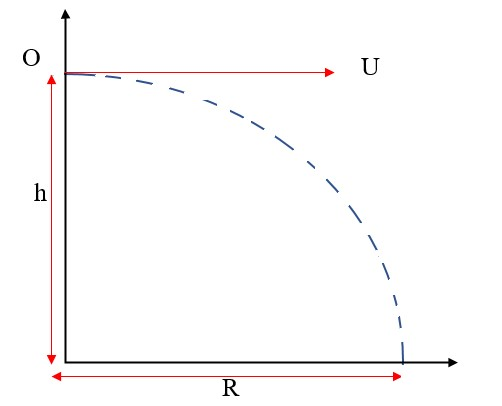The horizontal displacement 'R' at a time 't' is given by s = ut + ½at2
Taking u = u and a = 0 hence R = ut, is the horizontal displacement and h = ½gt2 is the vertical displacement.
NOTE: The time of flight is the same as the time of free fall.

Example 15
A ball is thrown from the top of a cliff 20m high with a horizontal velocity of 10m/s. Calculate,
a. The time taken by the ball to strike the ground.
b. The distance from the foot of the cliff to where the ball strikes the ground.
c. The vertical velocity at the time it strikes the ground. (take g=10m/s)
Solution

1. h = ½ gt2
20= ½ × 10 × t2
40 = 10t2
t2 = 40/10 = 4
t = 2 seconds
2. R = ut
= 10 × 2
= 20m

3. v = u + at = gt
= 2 × 10 = 20m/s